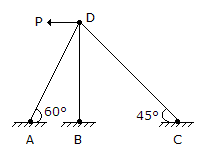# Civil Engineering - Theory of Structures - Discussion

Discussion Forum : Theory of Structures - Section 4 (Q.No. 16)
16.
The degree of indeterminacy of the frame in the given figure, iszero
1
2
3
Explanation:
No answer description is available. Let's discuss.
Discussion:
15 comments Page 1 of 2.

Muhammad usman said:   2 years ago
Since it is frame no of the internal loop is zero the reaction and the point where the load is acting like hinge then total indeterminacy is equal to external +internal.

External=number of reaction - equilibrium equation=6-3=3.
Internal=3c-rection realized by a hinge.

Here c=number of closed loop = 0.
Rr=reaction released by hinge = sum (m-1).

Here m is 3 and only one hinge so rr=3-1=2.
Internal indertminance =3*0-2=-2.

Total indeterminancy=3-2=1.

B.Singh said:   1 year ago
Degree of external indeterminacy = r - 3 = 6 - 3 = 3.
Degree of internal indeterminacy = m - (2j - 3) = 3 - (2*4 - 3) = -2,
Degree of static indeterminacy = External + Internal = 3 -2 = 1.

Alternatively,
Degree of static indeterminacy = (m + r) - 2j = (3 + 6) - 2 * 4 = 1.
(1)

EMAD ERNEST said:   8 years ago
We have x & y reaction at each of a, b & c giving 6 unknowns. Then the correct answer must be 3. But if the question was "Number of side ways" then the answer is 1.

Manoj Pakur said:   2 weeks ago
For frame;
3(m-j)+r-c
Here,
m=3
J=4
r=6
C=2
Solving, degree of indeterminacy = q.
(1)

Aman gupta said:   5 years ago
Its frame not a trus so formula= 3m+re-3j.

(3*3) +4- (3*4) =1.

Jaydip tiwari said:   2 years ago
I think M+r-2j is used for Truss not for FRAME.

Getasew said:   5 years ago
m = 3.
r = 6,
j = 4,
= 6+3-2*4.
= 1.

Omobola said:   2 years ago
I think it is (3*3) + 4- (3*4) = 0.

Abhik said:   5 years ago
M+r -2j is applied to truss.
(1)

Mamun said:   5 years ago
Here m+r-2j = 3+6-8 = 1.